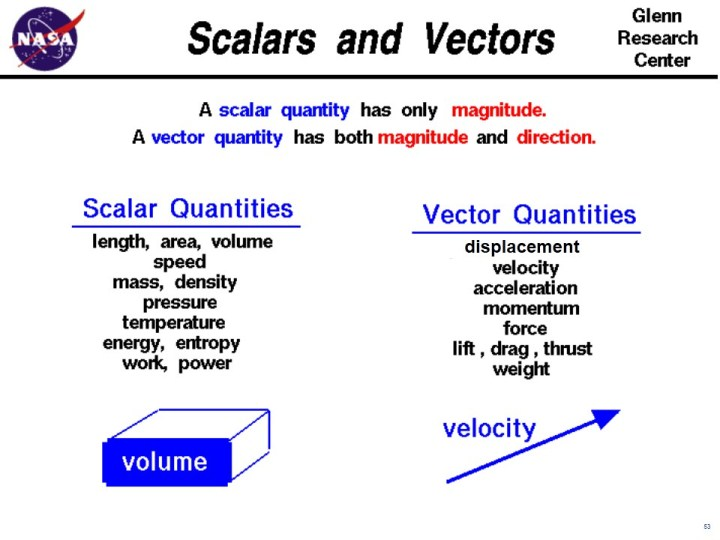+ Text Only Site
+ Non-Flash Version
+ Contact GlennTo better understand the science of propulsion it is necessary to use some mathematical ideas from vector analysis. Most people are introduced to vectors in high school or college, but for the elementary and middle school students, or the mathematically-challenged: DON'T PANIC!. There are many complex parts to vector analysis and we aren't going there. We are going to limit ourselves to the very basics. Vectors allow us to look at complex, multi-dimensional problems as a simpler group of one-dimensional problems. We will be concerned mostly with definitions The words are a bit strange, but the ideas are very powerful as you will see. If you want to find out a lot more about vectors you can download this report on vector analysis. Math and science were invented by humans to describe and understand the world around us. We live in a (at least) four-dimensional world governed by the passing of time and three space dimensions; up and down, left and right, and back and forth. We observe that there are some quantities and processes in our world that depend on the direction in which they occur, and there are some quantities that do not depend on direction. For example, the volume of an object, the three-dimensional space that an object occupies, does not depend on direction. If we have a 5 cubic foot block of iron and we move it up and down and then left and right, we still have a 5 cubic foot block of iron. On the other hand, the location, of an object does depend on direction. If we move the 5 cubic foot block 5 miles to the north, the resulting location is very different than if we moved it 5 miles to the east. Mathematicians and scientists call a quantity which depends on direction a vector quantity. A quantity which does not depend on direction is called a scalar quantity. Vector quantities have two characteristics, a magnitude and a direction. Scalar quantities have only a magnitude. When comparing two vector quantities of the same type, you have to compare both the magnitude and the direction. For scalars, you only have to compare the magnitude. When doing any mathematical operation on a vector quantity (like adding, subtracting, multiplying ..) you have to consider both the magnitude and the direction. This makes dealing with vector quantities a little more complicated than scalars. On the slide we list some of the physical quantities discussed in the Beginner's Guide to Aeronautics and group them into either vector or scalar quantities. Of particular interest, the forces which operate on a flying aircraft, the weight, thrust, and aerodynmaic forces, are all vector quantities. The resulting motion of the aircraft in terms of displacement, velocity, and acceleration are also vector quantities. These quantities can be determined by application of Newton's laws for vectors. The scalar quantities include most of the thermodynamic state variables involved with the propulsion system, such as the density, pressure, and temperature of the propellants. The energy, work, and entropy associated with the engines are also scalar quantities. Vectors have magnitude and direction, scalars only have magnitude. The fact that magnitude occurs for both scalars and vectors can lead to some confusion. There are some quantities, like speed, which have very special definitions for scientists. By definition, speed is the scalar magnitude of a velocity vector. A car going down the road has a speed of 50 mph. Its velocity is 50 mph in the northeast direction. It can get very confusing when the terms are used interchangeably! Another example is mass and weight. Weight is a force which is a vector and has a magnitude and direction. Mass is a scalar. Weight and mass are related to one another, but they are not the same quantity.` While Newton's laws describe the resulting motion of a solid, there are special equations which describe the motion of fluids, gases and liquids. For any physical system, the mass, momentum, and energy of the system must be conserved. Mass and energy are scalar quantities, while momentum is a vector quantity. This results in a coupled set of equations, called the Navier-Stokes equations, which describe how fluids behave when subjected to external forces. These equations are the fluid equivalent of Newton's laws of motion and are very difficult to solve and understand. A simplified version of the equations called the Euler equations can be solved for some fluids problems. Activities: Guided Tours Navigation ..Beginner's Guide Home Page+ Inspector General Hotline + Equal Employment Opportunity Data Posted Pursuant to the No Fear Act + Budgets, Strategic Plans and Accountability Reports + Freedom of Information Act + The President's Management Agenda + NASA Privacy Statement, Disclaimer, and Accessibility CertificationEditor: Nancy Hall NASA Official: Nancy Hall Last Updated: May 05 2015 + Contact Glenn